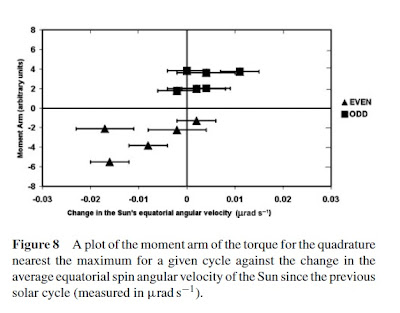## Wednesday, April 17, 2013

### A Confirmation of the VEJ Tidal-Torquing Model

UPDATED 30/04/2013

http://astroclimateconnection.blogspot.com.au/2013/04/further-confirmation-of-vej-tidal.html

The diagram below shows the solar sunspot number
(SSN) for solar cycles 13 through  to 23, plotted against
the net tangential torque of Jupiter acting upon the V-E tidal
bulge. The net tangential torque was obtained by adding
Jupiter's tangential torque at one V-E inferior conjunction
to Jupiter's tangential torque at the next V-E superior
conjunction. In this diagram, a positive net torque means
that the rotational speed of the Sun's equatorial convective
layer is sped-up and a negative net torque means that the
equatorial convective layer is slowed-down.

[N.B. The net torque curve has been smoother with a
5th and 7th order binomial filter to isolate low frequency
changes]

This diagram clearly shows that:

a) The net torque of Jupiter acting on the V-E tidal bulge
has a natural 22.4 year periodicity which matches the 22.1
year period of the solar Hale (magnetic) cycle.

b) the equatorial convective layers of the Sun are
sped-up during ODD solar cycles and slowed-down
during EVEN solar cycles.

Support for point b) is provided by the following
figure which was kindly sent to me as a private
communication by Paul Vaughan. Paul has allowed
me to use his graph here to support this post.

In this plot, Paul Vaughan has superposed the 22.1 year
JEV cycle onto Tlatov & Makarov's (2005) Figure 3.

Reference:
Tlatov, A.G.; & Makarov, V.I. (2005). 22-year variations
of the solar rotation, Large-scale Structures and their
Role in Solar Activity, ASP Conference Series,  Ed: K.
Sankarasubramanian, Matt Penn & Alexei Pevtsov,
Vol. 346,

http://www.solarstation.ru/TL/PDF/tl_22.pdf

The underlying figure from Tlatov & Makarov (2005) shows
the deviation of the surface rotation velocity of the Sun from
its average value at corresponding latitudes. These
measurements have been obtained from H-alpha observations
of the Sun.  In this diagram, the regions where rotation is
slower than normal are painted dark and the velocity is
averaged over the northern and southern hemispheres
(Note: The window for the spectral analysis was set to
12 years).

Paul Vaughan's graph clearly shows the 22.1 year
JEV cycle is almost perfectly synchronized in phase
with the changes in rotational velocity on the
surface of the Sun over a period of 100 years.

This is a remarkable result!

Further confirmation of point b) is given by the following figure
which compares the deviation of the surface rotation velocity
plot of Tlatov & Makarov (2005) with the monthly solar sunspot
number for cycles 13 through 23.

This plot  clearly shows that for all 11 solar cycles, the
deviation of the rotation velocity of the surface of the
Sun from its average value at corresponding latitudes,
speeds up during ODD solar cycles and slows down
during EVEN solar cycles.

This is a wonderful confirmation of my earlier results
published in  the blog post of November 23rd 2012.

It also indirectly confirms the result obtained in our 2008 solar paper:

Does a Spin–Orbit Coupling Between the Sun and the Jovian Planets Govern the Solar Cycle?

I. R. G. Wilson, B. D. Carter, and I. A. Waite
Publications of the Astronomical Society of
Australia, 2008, 25, 85–93.This graph from Wilson et al. 2008 (above) shows
the moment arm of the torque for the quadrature
Jupiter and Saturn nearest the maximum for a given
solar cycle, plotted against the change in the average
equatorial (spin) angular velocity of the Sun since
the previous solar cycle (measured in μrad s−1).
The equatorial (≤±15 deg) angular velocities published
by Javaraiah (2003) for cycles 12 to 23 have been
used to determine the changes in the Sun’s angular
velocity (since the previous cycle) for cycles 13 to 23.

What these graphs clearly show is that the Sun's equatorial
angular velocity increases in ODD solar cycles and decreases
in EVEN solar cycles, in a manner that is predicted by the
V-E-J Tidal-Torquing model.